# Sharpness: What is it and How it is Measured

View legacy documentation

## Measuring Sharpness

Sharpness determines the amount of detail an imaging system can reproduce. It is defined by the boundaries between zones of different tones or colors.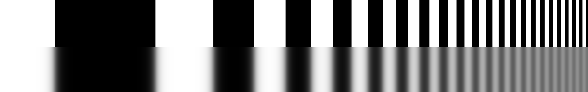Figure 1. Bar pattern: Original (upper half of figure) with lens degradation (lower half of figure)

In Figure 1, sharpness is illustrated as a bar pattern of increasing spatial frequency. The top portion of the figure is sharp and its boundaries are crisp; the lower portion is blurred and illustrates how the bar pattern is degraded after passing through a lens.

Note: All lenses blur images to some degree.

Sharpness is most visible on features like image edges (Figure 2) and can be measured by the edge (step) response.

Several methods are used for measuring sharpness that include the 10-90% rise distance technique, modulation transfer function (MTF), special and frequency domains, and slanted-edge algorithm.

### Rise Distance and Frequency Domain

A successful technique for measuring image sharpness is to use the “rise distance” of a tone or color edge.

With this technique, sharpness can be determined by the distance of a pixel level between 10% to 90% of its final value (also called 10-90% rise distance; see Figure 3).

Although rise distance is a good indicator of image sharpness, it also contains a strong limitation in that there is no easy method to calculate the rise distance of a complete imaging system from the rise distance of its components (i.e., lens, digital sensor, and software sharpening).

To sidestep this issue, measurements can be made in the frequency domain where frequency is measured in cycles or line pairs per distance (millimeters, inches, pixels, image height, and sometimes angle [degrees or milliradians]).

When measuring sharpness in the frequency domain, a complex signal (audio or image) can be created by combining signals consisting of pure tones (sine waves), which are characterized by a period or frequency (Figure 4).

Furthermore, frequency and spatial domains are related by the Fourier transform.

$$F(x)=\int_{-\infty}^{\infty}f(t)e^{-i\omega t}dt$$$$f(t)=\frac{1}{2\pi}\int_{-\infty}^{\infty}F(\omega)e^{i \omega t}d\omega$$

Where

Frequency = f = 1/Period (shorter period = higher frequency)t = timeω = 2πf

The better the system response at high frequencies (short periods), the more detail the system can convey (Figure 5). System response can be characterized by a frequency response curve, F(f).

Note: High frequencies correspond to fine detail.

### Modulation Transfer Function

The relative contrast at a given spatial frequency (output contrast/input contrast) is called Modulation Transfer Function (MTF), which is similar to the Spatial Frequency Response (SFR) and key to measuring sharpness. In Figure 6, MTF is illustrated as sine and bar patterns, an amplitude plot, and a contrast plot—each of which has spatial frequencies that increase continuously from left to right.

Note: Imatest uses SFR and MTF interchangeably. SFR is more commonly associated with complete system response, where MTF is commonly associated with the individual effects of a particular component. In other words, system SFR is equivalent to the product of the MTF of each component in the imaging system.

High spatial frequencies correspond to fine image detail. The response of photographic components (film, lenses, scanners, etc.) tends to “roll off” at high spatial frequencies. These components can be thought of as low-pass filters that pass low frequencies and attenuate high frequencies.

Figure 6 consists of upper, middle, and lower plots and are described as follows:

• Bar pattern, Sine pattern (upper plot) —The upper plot illustrates the original and sine patterns, sine pattern with lens blur, original bar pattern, and bar pattern with lens blur. (Note that lens blur causes contrast to drop at high spatial frequencies.)
• Amplitude (middle plot)—The middle plot displays the luminance (“modulation”  V in the MTF Equation section) of the bar pattern with lens blur (see red curve in Figure 6). The modulation of the sine pattern, which consists of pure frequencies, is used to calculate MTF. (Note that contrast decreases at high spatial frequencies.)
• MTF % (lower plot)—The lower plot shows the corresponding sine pattern contrast (see blue curve; represents MTF), which also is defined in the MTF Equation section. By definition, the low frequency MTF limit is always 1 (100%). In Figure 6, the MTF is 50% at 61 lp/mm and 10% at 183 lp/mm. (Note that both frequency and MTF are displayed on logarithmic scales with exponential notation [100 = 1; 101 = 10; 102 = 100, etc.]; amplitude is displayed on a linear scale. The MTF of a complete imaging system is the product of the MTF of its individual components.)

#### MTF Equation

The equation for MTF is derived from the sine pattern contrast C(f) at spatial frequency f, where

$$C(f)=\frac{V_{max}-V_{min}}{V_{max}+V_{min}}$$

for luminance (“modulation”) V.

Note$$MTF(f)=100\% \times\frac{C(f)}{C(0)}$$ normalizes MTF to 100% at low spatial frequencies.

To normalize MTF at low spatial frequencies, a test chart must have a low-frequency reference. The low-frequency reference is satisfied by large light and dark areas in slanted edges and by features in most patterns used by Imatest, but not satisfied by lines and grids. For systems where sharpening can be controlled, the recommended primary MTF calculation is the slanted-edge, which uses a mathematical operation known as the Fourier transform. In other words, MTF is the Fourier transform of the impulse response (i.e., response to a narrow line) that is the derivative (d/dx or d/dy) of the edge response.

Note: Imatest recommends the star or web patterns if the sharpening of the image signal processor cannot be disabled.

Traditional resolution measurements involve observing an image of a bar pattern, often contained within the USAF 1951 chart (Figure 7) and looking for the highest spatial frequency (lp/mm) where bar patterns are visibly distinct. Traditional measurements (also called vanishing resolution measurement) correspond to an MTF of roughly 10-20%. Because the vanishing resolution measurement is the spatial frequency where image information disappears, it is strongly dependent on observer bias and is a poor indicator of image sharpness.

Note: The USAF 1951 chart is poorly suited for computer analysis because it uses space inefficiently and its bar triplets lack a low frequency reference. Furthermore, small changes in the chart position (sampling phase) can cause the chart bars to appear or disappear. The chart also is prone to aliasing where small changes of position can cause the appearance of its bars to change when they shift from being in phase or out of phase with the pixel array.

Good indicators of image sharpness are spatial frequencies where MTF is 50% of its low frequency value (MTF50) or 50% of its peak value (MTF50P). MTF50 and MTF50P are good parameters for comparing the sharpness of different cameras and lenses for two reasons:

1. Image contrast is half its low frequency or peak value thus detail is still quite visible. (The eye is relatively insensitive to detail at spatial frequencies where MTF is 10% or less.)
2. The response of most cameras falls off rapidly in the vicinity of MTF50 and MTF50P. MTF50P is a better metric for strongly sharpened cameras that have “halos” near edges and corresponding peaks in their MTF response.

Note: Acutance, MTF at stable low frequencies, and MTF Area are also good indicators of image sharpness.

Although MTF can be estimated directly from images of sine patterns (using Rescharts,Log FrequencyLog F-Contrast, and Star Chart), a sophisticated technique based on the ISO 12233 provides more accurate and repeatable results and uses space more efficiently. (See Slanted-edge versus Siemens Star for more details.)  A slanted-edge image, described below, can be photographed, then analyzed by Imatest SFRSFRplus, or eSFR ISO.

### Spatial Frequency Units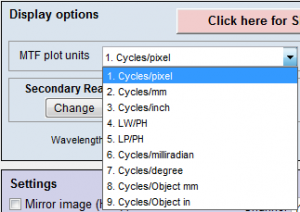Figure 8. Spatial frequency units are selected in the Settings or More settings windows of SFR and Rescharts modules (SFRplus, eSFR ISO, Star, etc.).

Most readers will be familiar with temporal frequency. The frequency of a sound—measured in Cycles/Second or Hertz—is closely related to its perceived pitch. The frequencies of radio transmissions (measured in kilohertz, megahertz, and gigahertz) are also familiar. Spatial frequency is similar because it is measured in cycles (or line pairs) per distance instead of time. Spatial frequency response is closely analogous to temporal (e.g., audio) frequency response. The more extended the response, the more detail can be conveyed.

Spatial frequency units should be selected from the Settings or More settings windows of SFR and Rescharts modules (SFRplus, eSFR ISO, Star, etc. See Figure 8) and is the measurement intended to determine how much detail a camera can reproduce or how well the pixels are utilized.

Past film camera lens tests used line pairs per millimeter (lp/mm), which worked well for comparing lenses because most 35mm film cameras have the same 24 x 36mm picture size. Yet, digital sensor sizes vary widely—from under 5mm diagonal in camera phones to 43mm diagonal for full-frame DSLRs to an even larger diagonal for medium format backs. For this reason, line widths per picture height (LW/PH) is recommended for measuring the total detail a camera can reproduce. Note that LW/PH is equal to 2 * lp/mm * (picture height in mm).

Another useful spatial frequency unit is cycles per pixel (C/P), which gives an indication of how well individual pixels are utilized. There is no need to use actual distances (millimeters or inches) with digital cameras, although such measurements are available (Table 1).

Table 1. Summary of spacial frequency units with equations that refer to MTF in selected frequency units.

 MTF Unit Application Equation Cycles/Pixel (C/P) Shows how well pixels are utilized. Nyquist frequency fNyq is always 0.5 C/P. Cycles/Distance (cycles/mm or cycles/inch) Cycles per distance on the sensor. Pixel spacing or pitch must be entered. Popular for comparing resolution in the old days of standard film formats (e.g., 24x36mm for 35mm film). $$\displaystyle \frac{MTF(C/P)}{\text{pixel pitch}}$$ Line Widths/Picture Height (LW/PH) Measures overall image sharpness. This is the best unit for comparing the performance of cameras with different sensor sizes and pixel counts. Line Widths is traditional for TV measurements. Note that 1 Cycle = 1 Line Pair (LP) = 2 Line Widths (LW). $$2 \times MTF\bigl(\frac{LP}{PH}\bigr)$$ ; $$2 \times MTF\bigl(\frac{C}{P}\bigr) times PH$$ Line Pairs/Picture Height (LP/PH) Measures overall image sharpness. Differs from LW/PH by a factor of 2. Used by dpreview.com. $$MTF\bigl(\frac{LW}{PH}\bigr) / 2$$ ; $$MTF\bigl(\frac{C}{P}\bigr) times PH$$ Cycles/milliradian Angular frequencies. Pixel spacing or pitch must be entered. Focal length (FL) in mm is usually included in EXIF data in commercial image files. If it isn’t available it must be entered manually, typically in the EXIF parameters region at the bottom of the settings window. If pixel spacing or focal length is missing, units will default to Cycles/Pixel. Cycles/degree is useful for comparing camera systems to the human eye, which has an MTF50 of roughly 20 Cycles/Degree (depending on the individual’s eyesight and illumination). FL can be calculated from the simple lens equation, $$1/FL = 1/s_1 + 1/s_2$$, where s1 is the lens-to-chart distance, s2 is the lens-to-sensor distance, and magnification $$|M| = s_2/s_1$$. $$FL = s_1/(1-1/M)$$. $$\displaystyle 0.001 \times MTF\bigl(\frac{\text{cycles}}{\text{mm}}\bigr) \times FL(\text{mm})$$ Cycles/degree $$\displaystyle \frac{\pi}{180} \times MTF\bigl(\frac{\text{cycles}}{\text{mm}}\bigr) \times FL(\text{mm})$$ Cycles/object mm Cycles/object in Cycles per distance on the object being photographed (what many people think of as the subject). Pixel spacing and magnification must be entered. Important when the system specification references the object being photographed (for example, if cracks of a certain width need to be detected). $$\displaystyle MTF\bigl( \frac{\text{Cycles}}{\text{Distance}} \bigr) \times |\text{Magnification}|$$ Line Widths/Crop Height Line Pairs/Crop Height Primarily used for testing when the active chart height (rather than the total image height) is significant. PH = Picture Height in pixels. FL(mm) = Lens focal length in mm.  Pixel pitch = distance per pixel = 1/(pixels per distance).   Note: Different units scale differently with image sensor and pixel size.

#### Summary Metrics

Several summary metrics are derived from MTF curves to characterize overall performance. These metrics are used in a number of displays, including secondary readouts in the SFR/SFRplus/eSFR ISO Edge/MTF plot (see Imatest Slanted-Edge Results) and in the SFRplus 3D maps.

Note: See Summary Metrics Derived from MTF Curve for additional information.

### Slanted-Edge Measurement for Spatial Frequency Response

Several Imatest modules measure MTF using the slanted-edge technique and include:

• Slanted-edge test charts that may be purchased from Imatest or created with Imatest Test Charts. Charts that employ automatic detection (SFRpluseSFR ISOSFRreg, or Checkerboard) are recommended.
• Briefly, the ISO 12233 slanted-edge method calculates MTF by finding the average edge (4X oversampled using a clever binning algorithm), differentiating it (to obtain the Line Spread Function (LSF)), and taking the absolute value of the Fourier transform of the LSF. The algorithm is described in detail here.

#### Why a Slanted Edge?

MTF results for pure vertical or horizontal edges are highly dependent on sampling phase (the relationship between the edge and the pixel locations), and hence can vary from one run to the next depending on the precise (sub-pixel) edge position. The edge is slanted so MTF is calculated from the average of many sampling phases, which makes results much more stable and robust (Figure 9).

#### Edge Contrast

Edge Contrast should be limited to 10:1 at the most, and a 4:1 edge contrast is generally recommended. The reason is that high contrast edges (>10:1, such as found in the old ISO 12233:2000 chart) can cause saturation or clipping, resulting in edges with sharp corners that exaggerate MTF measurements.

• Most efficient use of space, which makes it possible to create a detailed map of MTF response
• Fast, automated region detection in SFRplus, eSFR ISO, SFRreg, and Checkerboard
• Fast calculations
• Relatively insensitive to noise (highly immune if noise reduction is applied)
• Compliant with the ISO 12233 standard, whose “binning” (super-resolution) algorithm allows MTF to be measured above the Nyquist frequency (0.5 C/P)
• The best pattern for manufacturing testing
• May give optimistic results in systems with strong sharpening and noise reduction (i.e., can be fooled by signal processing, especially with high contrast [≥ 10:1] edges)
• Gives inconsistent results in systems with extreme aliasing (strong energy above the Nyquist frequency), especially with small regions
• Sensitive to sharpening, especially for high contrast (≥10:1) edges; less sensitive for low contrast edges (≤2:1)
• Least sensitive to software noise reduction.

Note: Imatest Master can calculate MTF for edges of virtually any angle, though exact vertical, horizontal, and 45° should be avoided because of sampling phase sensitivity.

#### Slanted-Edge Modules

Imatest Slanted-Edge Modules include SFR, SFRplus, eSFR ISO, Checkerboard, and SFRreg (see Table 2 and Sharpness Modules for details).

Note: See “How to test lenses with Imatest” for a good summary of how to measure MTF using SFRplus or eSFR ISO.

Table 2. Brief summary of Imatest slanted-edge modules.

ModuleDescriptionExamples
SFR
• Measures MTF from slanted edges in a variety of charts and wherever there is a clean edge; region selection is manual.
• Two useful regions from the old ISO-12233:2000 chart are indicated by red and blue arrows (see top, far-right image in the Examples column).
• A typical region (a crop of a vertical edge slanted about 5.7 degrees) is used to calculate horizontal MTF response (see lower, far-right image in the Examples column).SFRplus
• Measures MTF and other image quality parameters from Imatest SFRplus chart (recommended) or created using Imatest Test Charts (a wide-body printer, advanced printing skills, and knowledge of color management required).

• Offers numerous advantages over the old ISO 12233:2000 test chart: automatic feature detection, lower contrast for improved accuracy, more edges (less wasted space) for a detailed map of MTF over the image surface.

• Measurements are ISO-compliant; includes automatic region detection.eSFR ISO
• Measures MTF and other image quality parameters using an enhanced version of the ISO 12233:2014 and 2017 Edge SFR (E-SFR) test chart

• Has slightly less spatial detail than SFRplus, but much more noise detail.

• Includes automatic region detection.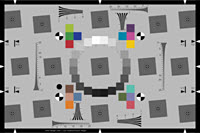Checkerboard
• Sensitive to framing, making it ideal for through-focus tests.

• Provides precise distortion calculations.

• Includes automatic region detection.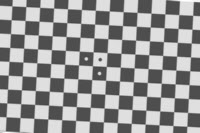SFRreg Several individual charts are typically placed around the image field; works with:
• Extreme fisheye lenses (>180º)

• Charts at different distances to test focus and depth of field.

• Extreme high resolution (>36MP) cameras, large fields of view, and large distances.

• Includes automatic region detection.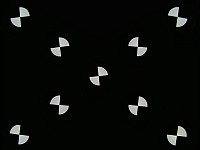#### Slanted-Edge Algorithm

The MTF calculation is derived from ISO standard 12233. Some details are contained in The Imatest calculation contains a number of enhancements, listed below. The original ISO calculation is performed when the ISO standard SFR checkbox in the SFR input dialog box is checked. See below for additional information:

• The cropped image is linearized; i.e., the pixel levels are adjusted to remove the gamma encoding applied by the camera. (Gamma is adjustable with a default of 0.5).
• The edge locations for the red, green, blue, and luminance channels:
Y=0.3R+0.59G+0.11B or 0.2125R+0.7154G+0.0721B
are determined for each scan line (horizontal lines in the above image).
• A second order fit to the edge is calculated for each channel using polynomial regression. The second order fit removes the effects of lens distortion. In the above image, the equation would have the form:
x=a0+a1y+a2y2
• Depending on the value of the fractional part
fp = xi – int(xi )
of the second order fit at each scan line, the shifted edge is added to one of four bins:
bin 1 if 0 ≤ fp < 0.25
bin 2 if 0.25 ≤ fp < 0.5
bin 3 if 0.5 ≤ fp < 0.75
bin 4 if 0.75 ≤ fp < 1

Note: The bin mentioned in the previous equation does not depend on the detected edge location.

• The four bins are combined to calculate an averaged 4x oversampled edge. This allows analysis of spatial frequencies beyond the normal Nyquist frequency.
• The derivative (d/dx) of the averaged 4x oversampled edge is calculated. A windowing function is applied to force the derivative to zero at its limits.
• MTF is the absolute value of the Fourier transform (FFT) of the windowed derivative.

Note: Origins of Imatest slanted-edge SFR calculations were adapted from a Matlab program, sfrmat, which was written by Peter Burns to implement the ISO 12233:2000 standard. Imatest’s SFR calculation incorporates numerous improvements, including improved edge detection, better handling of lens distortion, and better noise immunity. The original Matlab code is available here. In comparing sfrmat results with Imatest, tonal response is assumed to be linear; i.e., gamma = 1 if no OECF (tonal response curve) file is entered into sfrmat. Since the default value of gamma in Imatest is 0.5, which is typical of digital cameras, you must set gamma to 1 to obtain good agreement with sfrmat.

Several related techniques affect sharpness results, including:

## ISO 12233: Differences for Imatest Calculations

Several differences between ISO 12233 calculations and those used by Imatest can be found here. Note that Additional calculation details can be found in the Peter Burns links (below).